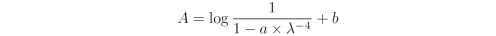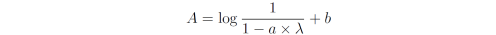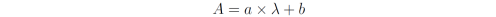### Absorption spectrum of scattering solution

If a sample contains particles smaller than the wavelength of light, such as aggregates, a precipitate, colloids, etc., incoming light will be scattered elastically from the solution. This is known as Rayleigh scatter. Rayleigh scatter will influence the measured absorption spectrum since the scattered light will not reach the detector of the absorption spectrometer and, thus, will be interpreted as absorbed light by the instrument.

The intensity of Rayleigh scatter is proportional to l-4, i.e. scattering is more intense towards the blue end of the spectrum. The absorption spectrum of a purely Rayleigh scattering solution becomes:where I0 is the intensity of the incoming light, I is the intensity hitting the detector, A0 is a constant and c is a constant of proportionality. This function is plotted in the figure below for A0 = 0 and c = 109:Figure. Absorption spectrum of purely scattering solution.

As a result, absorption spectra that appear to have slightly bad baselines in the red end of the spectrum (where the sample does not absorb), may in fact have very bad baselines where the sample absorb in the blue end of the spectrum - if the scatter is not properly subtracted by the absorption baseline. In my experience, this is often an unrecognized large source of error in quantitative optical spectroscopy.

### Subtract scatter from absorption spectra using a|e

a|e provides a fitting routine that estimates the scatter-function of an absorption spectrum. The fitted scatter function can then be subtracted from the absorption spectrum in order to correct for bad baselines resulting from scattering.

Note that this is not the most optimal way to correct absorption spectra. Ideally, a good baseline should be acquired on the day of experiment.

OBS: The background tool was changed in version 2.0. The scatter function is now located at: Tools->Background Tools->Subtract scatter

#### Guide for subtracting scatter in a|e versions 1.x

1. Make sure the 'Background subtraction' toggle button is activated. This will make the boxes and buttons associated with baseline subtraction visible.

2. For clarity, only the spectrum under investigation is plotted here (D_abs). Select the Data cursor tool from the toolbar.

3. The data cursor tool is used to define the data range used in fitting the background-scatter function. Use the Alt key to position multiple data cursor points on the selected spectrum.

This is how the data cursor positions define the data range to fit:
The two data cursor points located farthest to the right (i.e. the red part of the spectrum) define the main data interval. This interval should not overlap with any absorption bands in the spectrum but merely define the red part of the spectrum where the absorption is known to be 0 (in absence of scatter).

Any additionally selected points ('3' below) define single data points that are added to the data range. These additional points are positioned at higher-energy wavelengths (i.e. to the left of the lowest energy absorption band) where the absorption spectrum is known to be A = 0.

In general, the purpose is to select as many data points as possible where the absorption is known to be A = 0.

4. Press the 'Fit' button located below the 'Absorption baselines' data listbox. The fitted scatter-function is now added to the Absorption baselines listbox and, if selected, plotted in the absorption window in black.

5. Since only a small spectral region can usually be used in the fitting, and the scatter function increases dramatically at lower wavelength as shown in the first figure above, this fitting procedure could well result in a fit that does not at all represent scatter. It is thus important that the fitted scatter baseline is below the absorption spectrum throughout the entire spectrum which must be checked manually.

5b. If the fit is bad at higher energies, you can try to use a fit function with a less steep wavelength dependency. Scroll to the "Alternate" or "Linear" fit functions (do this next to the Fit button below the listbox) and use one of these scatter functions instead. The three possible scatter fit functions are:

"Scatter":"Alternate":"Linear":While the latter two functions are not physically meaningful they do not depend just as dramatically on the wavelength. This is an advantage when a narrow data range is available for fitting (i.e. the sample absorbs throughout the visible region). The fit using these functions could thus provide a better estimate of the actual scatter function than that fitted using a lambda^-4 dependency. Below is shown each of the fits obtained using the three different scatter functions:

"Scatter"

"Alternate"

6. In order to subtract the newly produced scatter baseline press the subtract button located below the 'Absorption spectra' data listbox.

This will open a dialog box. In the right listbox you select the baseline to subtract from all absorption spectra selected in the left listbox. Press OK.